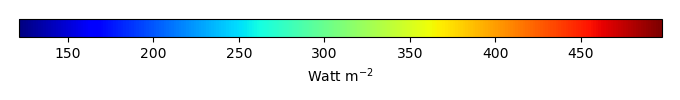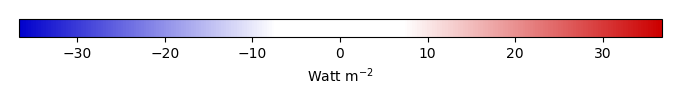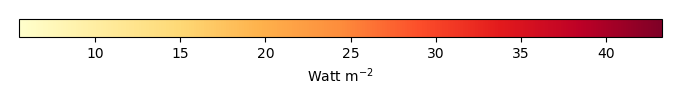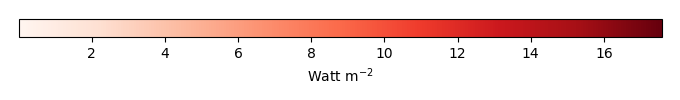# Mean State

Period Mean (original grids) [Watt m-2]
Model Period Mean (intersection) [Watt m-2]
Model Period Mean (complement) [Watt m-2]
Benchmark Period Mean (intersection) [Watt m-2]
Benchmark Period Mean (complement) [Watt m-2]
Bias [Watt m-2]
RMSE [Watt m-2]
Phase Shift [months]
Bias Score 
RMSE Score 
Seasonal Cycle Score 
Spatial Distribution Score 
Interannual Variability Score 
Overall Score 
Benchmark [-] 453.
CLM4 [-] 457. 457. 0.00 453. 460. 4.84 13.4 1.57 0.54 0.38 0.77 0.95 0.74 0.63
CLM4.5 [-] 461. 461. 0.00 453. 460. 8.90 16.0 1.54 0.44 0.38 0.77 0.96 0.73 0.61
CLM5 [-] 462. 462. 0.00 453. 460. 10.0 15.8 1.71 0.36 0.38 0.75 0.99 0.72 0.60
Period Mean (original grids) [Watt m-2]
Model Period Mean (intersection) [Watt m-2]
Model Period Mean (complement) [Watt m-2]
Benchmark Period Mean (intersection) [Watt m-2]
Benchmark Period Mean (complement) [Watt m-2]
Bias [Watt m-2]
RMSE [Watt m-2]
Phase Shift [months]
Bias Score 
RMSE Score 
Seasonal Cycle Score 
Spatial Distribution Score 
Interannual Variability Score 
Overall Score 
Benchmark [-] 471.
CLM4 [-] 478. 479. 0.00 471. 456. 6.98 20.8 0.812 0.60 0.47 0.94 0.98 0.79 0.71
CLM4.5 [-] 483. 484. 0.00 471. 456. 12.0 23.5 0.808 0.51 0.48 0.93 1.0 0.78 0.70
CLM5 [-] 485. 485. 0.00 471. 456. 13.4 23.9 0.875 0.50 0.47 0.93 1.0 0.76 0.69
Period Mean (original grids) [Watt m-2]
Model Period Mean (intersection) [Watt m-2]
Model Period Mean (complement) [Watt m-2]
Benchmark Period Mean (intersection) [Watt m-2]
Benchmark Period Mean (complement) [Watt m-2]
Bias [Watt m-2]
RMSE [Watt m-2]
Phase Shift [months]
Bias Score 
RMSE Score 
Seasonal Cycle Score 
Spatial Distribution Score 
Interannual Variability Score 
Overall Score 
Benchmark [-] 443.
CLM4 [-] 446. 446. 0.00 443. 444. 2.95 17.0 0.787 0.59 0.52 0.91 0.93 0.67 0.69
CLM4.5 [-] 451. 451. 0.00 443. 444. 8.04 19.1 0.838 0.51 0.52 0.91 0.94 0.68 0.68
CLM5 [-] 450. 449. 0.00 443. 444. 6.37 18.0 0.975 0.56 0.51 0.89 0.96 0.64 0.68
Period Mean (original grids) [Watt m-2]
Model Period Mean (intersection) [Watt m-2]
Model Period Mean (complement) [Watt m-2]
Benchmark Period Mean (intersection) [Watt m-2]
Benchmark Period Mean (complement) [Watt m-2]
Bias [Watt m-2]
RMSE [Watt m-2]
Phase Shift [months]
Bias Score 
RMSE Score 
Seasonal Cycle Score 
Spatial Distribution Score 
Interannual Variability Score 
Overall Score 
Benchmark [-] 276.
CLM4 [-] 257. 257. 0.00 275. 292. -15.2 24.6 0.0720 0.78 0.75 0.99 0.97 0.79 0.84
CLM4.5 [-] 259. 259. 0.00 275. 292. -13.8 23.1 0.0685 0.80 0.76 1.0 0.96 0.77 0.84
CLM5 [-] 265. 265. 0.00 275. 292. -7.59 19.0 0.0691 0.86 0.78 1.0 0.97 0.77 0.86
Period Mean (original grids) [Watt m-2]
Model Period Mean (intersection) [Watt m-2]
Model Period Mean (complement) [Watt m-2]
Benchmark Period Mean (intersection) [Watt m-2]
Benchmark Period Mean (complement) [Watt m-2]
Bias [Watt m-2]
RMSE [Watt m-2]
Phase Shift [months]
Bias Score 
RMSE Score 
Seasonal Cycle Score 
Spatial Distribution Score 
Interannual Variability Score 
Overall Score 
Benchmark [-] 456.
CLM4 [-] 450. 450. 0.00 453. 465. -0.720 9.44 2.02 0.36 0.37 0.69 0.99 0.67 0.57
CLM4.5 [-] 452. 452. 0.00 453. 465. 0.402 9.67 2.00 0.36 0.37 0.69 0.99 0.67 0.58
CLM5 [-] 456. 456. 0.00 453. 465. 4.81 10.5 2.03 0.31 0.38 0.68 0.99 0.67 0.57
Period Mean (original grids) [Watt m-2]
Model Period Mean (intersection) [Watt m-2]
Model Period Mean (complement) [Watt m-2]
Benchmark Period Mean (intersection) [Watt m-2]
Benchmark Period Mean (complement) [Watt m-2]
Bias [Watt m-2]
RMSE [Watt m-2]
Phase Shift [months]
Bias Score 
RMSE Score 
Seasonal Cycle Score 
Spatial Distribution Score 
Interannual Variability Score 
Overall Score 
Benchmark [-] 306.
CLM4 [-] 302. 302. 0.00 306. 329. -3.13 11.9 0.0220 0.93 0.86 1.0 0.98 0.89 0.92
CLM4.5 [-] 303. 303. 0.00 306. 329. -2.69 11.8 0.0220 0.94 0.86 1.0 0.98 0.89 0.92
CLM5 [-] 309. 309. 0.00 306. 329. 3.73 11.7 0.0258 0.92 0.87 1.0 0.98 0.89 0.92
Period Mean (original grids) [Watt m-2]
Model Period Mean (intersection) [Watt m-2]
Model Period Mean (complement) [Watt m-2]
Benchmark Period Mean (intersection) [Watt m-2]
Benchmark Period Mean (complement) [Watt m-2]
Bias [Watt m-2]
RMSE [Watt m-2]
Phase Shift [months]
Bias Score 
RMSE Score 
Seasonal Cycle Score 
Spatial Distribution Score 
Interannual Variability Score 
Overall Score 
Benchmark [-] 362.
CLM4 [-] 356. 355. 0.00 361. 395. -3.37 14.5 0.126 0.86 0.83 0.99 1.0 0.77 0.88
CLM4.5 [-] 356. 356. 0.00 361. 395. -2.66 14.3 0.114 0.87 0.83 0.99 1.0 0.77 0.88
CLM5 [-] 361. 360. 0.00 361. 395. 1.74 14.5 0.198 0.87 0.83 0.99 1.0 0.75 0.88
Period Mean (original grids) [Watt m-2]
Model Period Mean (intersection) [Watt m-2]
Model Period Mean (complement) [Watt m-2]
Benchmark Period Mean (intersection) [Watt m-2]
Benchmark Period Mean (complement) [Watt m-2]
Bias [Watt m-2]
RMSE [Watt m-2]
Phase Shift [months]
Bias Score 
RMSE Score 
Seasonal Cycle Score 
Spatial Distribution Score 
Interannual Variability Score 
Overall Score 
Benchmark [-] 450.
CLM4 [-] 451. 452. 0.00 450. 463. 4.42 18.4 0.476 0.62 0.56 0.96 0.97 0.69 0.73
CLM4.5 [-] 455. 456. 0.00 450. 463. 8.17 19.8 0.491 0.57 0.56 0.96 0.98 0.69 0.72
CLM5 [-] 457. 458. 0.00 450. 463. 10.2 20.0 0.673 0.56 0.57 0.94 0.99 0.65 0.71
Period Mean (original grids) [Watt m-2]
Model Period Mean (intersection) [Watt m-2]
Model Period Mean (complement) [Watt m-2]
Benchmark Period Mean (intersection) [Watt m-2]
Benchmark Period Mean (complement) [Watt m-2]
Bias [Watt m-2]
RMSE [Watt m-2]
Phase Shift [months]
Bias Score 
RMSE Score 
Seasonal Cycle Score 
Spatial Distribution Score 
Interannual Variability Score 
Overall Score 
Benchmark [-] 275.
CLM4 [-] 265. 265. 0.00 275. 280. -9.37 20.3 0.0384 0.86 0.75 1.0 0.97 0.87 0.87
CLM4.5 [-] 265. 265. 0.00 275. 280. -9.31 19.2 0.103 0.86 0.76 0.99 0.97 0.85 0.87
CLM5 [-] 273. 273. 0.00 275. 280. -1.59 15.1 0.0657 0.92 0.79 1.0 0.98 0.85 0.89
Period Mean (original grids) [Watt m-2]
Model Period Mean (intersection) [Watt m-2]
Model Period Mean (complement) [Watt m-2]
Benchmark Period Mean (intersection) [Watt m-2]
Benchmark Period Mean (complement) [Watt m-2]
Bias [Watt m-2]
RMSE [Watt m-2]
Phase Shift [months]
Bias Score 
RMSE Score 
Seasonal Cycle Score 
Spatial Distribution Score 
Interannual Variability Score 
Overall Score 
Benchmark [-] 448.
CLM4 [-] 447. 447. 0.00 450. 423. -4.99 14.2 0.331 0.77 0.72 0.97 1.0 0.77 0.83
CLM4.5 [-] 448. 448. 0.00 450. 423. -4.69 14.4 0.322 0.76 0.72 0.97 1.0 0.77 0.82
CLM5 [-] 450. 450. 0.00 450. 423. -2.94 14.0 0.347 0.78 0.71 0.97 1.0 0.74 0.82
Period Mean (original grids) [Watt m-2]
Model Period Mean (intersection) [Watt m-2]
Model Period Mean (complement) [Watt m-2]
Benchmark Period Mean (intersection) [Watt m-2]
Benchmark Period Mean (complement) [Watt m-2]
Bias [Watt m-2]
RMSE [Watt m-2]
Phase Shift [months]
Bias Score 
RMSE Score 
Seasonal Cycle Score 
Spatial Distribution Score 
Interannual Variability Score 
Overall Score 
Benchmark [-] 364.
CLM4 [-] 357. 357. 0.00 362. 385. -2.12 11.7 0.237 0.85 0.79 0.98 1.0 0.83 0.88
CLM4.5 [-] 356. 356. 0.00 362. 385. -2.72 10.9 0.215 0.86 0.80 0.99 1.0 0.84 0.88
CLM5 [-] 361. 361. 0.00 362. 385. 1.58 10.8 0.513 0.86 0.81 0.97 1.0 0.83 0.88
Period Mean (original grids) [Watt m-2]
Model Period Mean (intersection) [Watt m-2]
Model Period Mean (complement) [Watt m-2]
Benchmark Period Mean (intersection) [Watt m-2]
Benchmark Period Mean (complement) [Watt m-2]
Bias [Watt m-2]
RMSE [Watt m-2]
Phase Shift [months]
Bias Score 
RMSE Score 
Seasonal Cycle Score 
Spatial Distribution Score 
Interannual Variability Score 
Overall Score 
Benchmark [-] 397.
CLM4 [-] 372. 372. 0.00 376. 406. -2.67 16.3 0.474 0.72 0.67 0.95 1.0 0.72 0.79
CLM4.5 [-] 373. 373. 0.00 376. 406. -1.39 16.7 0.478 0.71 0.66 0.95 1.0 0.72 0.78
CLM5 [-] 377. 377. 0.00 376. 406. 1.88 16.1 0.535 0.71 0.67 0.94 1.0 0.70 0.78
Period Mean (original grids) [Watt m-2]
Model Period Mean (intersection) [Watt m-2]
Model Period Mean (complement) [Watt m-2]
Benchmark Period Mean (intersection) [Watt m-2]
Benchmark Period Mean (complement) [Watt m-2]
Bias [Watt m-2]
RMSE [Watt m-2]
Phase Shift [months]
Bias Score 
RMSE Score 
Seasonal Cycle Score 
Spatial Distribution Score 
Interannual Variability Score 
Overall Score 
Benchmark [-] 464.
CLM4 [-] 452. 452. 0.00 465. 441. -12.5 21.9 0.404 0.73 0.75 0.97 1.0 0.66 0.81
CLM4.5 [-] 452. 452. 0.00 465. 441. -12.5 21.9 0.405 0.73 0.75 0.97 1.0 0.66 0.81
CLM5 [-] 454. 454. 0.00 465. 441. -11.1 21.4 0.446 0.75 0.74 0.96 0.99 0.65 0.81
Period Mean (original grids) [Watt m-2]
Model Period Mean (intersection) [Watt m-2]
Model Period Mean (complement) [Watt m-2]
Benchmark Period Mean (intersection) [Watt m-2]
Benchmark Period Mean (complement) [Watt m-2]
Bias [Watt m-2]
RMSE [Watt m-2]
Phase Shift [months]
Bias Score 
RMSE Score 
Seasonal Cycle Score 
Spatial Distribution Score 
Interannual Variability Score 
Overall Score 
Benchmark [-] 278.
CLM4 [-] 270. 270. 0.00 277. 289. -8.21 17.8 0.0696 0.85 0.78 1.0 0.96 0.79 0.86
CLM4.5 [-] 270. 270. 0.00 277. 289. -7.99 17.1 0.0688 0.85 0.79 1.0 0.96 0.78 0.86
CLM5 [-] 277. 276. 0.00 277. 289. -1.61 14.2 0.0692 0.90 0.81 1.0 0.97 0.78 0.88
Period Mean (original grids) [Watt m-2]
Model Period Mean (intersection) [Watt m-2]
Model Period Mean (complement) [Watt m-2]
Benchmark Period Mean (intersection) [Watt m-2]
Benchmark Period Mean (complement) [Watt m-2]
Bias [Watt m-2]
RMSE [Watt m-2]
Phase Shift [months]
Bias Score 
RMSE Score 
Seasonal Cycle Score 
Spatial Distribution Score 
Interannual Variability Score 
Overall Score 
Benchmark [-] 444.
CLM4 [-] 444. 444. 0.00 442. 455. 6.79 17.1 0.558 0.51 0.56 0.94 0.84 0.76 0.69
CLM4.5 [-] 443. 443. 0.00 442. 455. 4.68 15.1 0.543 0.57 0.57 0.94 0.92 0.75 0.72
CLM5 [-] 445. 445. 0.00 442. 455. 6.86 15.5 0.625 0.53 0.58 0.94 0.93 0.73 0.71
Period Mean (original grids) [Watt m-2]
Model Period Mean (intersection) [Watt m-2]
Model Period Mean (complement) [Watt m-2]
Benchmark Period Mean (intersection) [Watt m-2]
Benchmark Period Mean (complement) [Watt m-2]
Bias [Watt m-2]
RMSE [Watt m-2]
Phase Shift [months]
Bias Score 
RMSE Score 
Seasonal Cycle Score 
Spatial Distribution Score 
Interannual Variability Score 
Overall Score 
Benchmark [-] 386.
CLM4 [-] 380. 380. 0.00 384. 429. -1.49 9.00 0.148 0.90 0.85 0.99 1.0 0.89 0.91
CLM4.5 [-] 381. 381. 0.00 384. 429. -0.369 9.36 0.145 0.90 0.84 0.99 1.0 0.90 0.91
CLM5 [-] 387. 387. 0.00 384. 429. 5.60 10.2 0.275 0.87 0.85 0.98 1.0 0.89 0.91
Period Mean (original grids) [Watt m-2]
Model Period Mean (intersection) [Watt m-2]
Model Period Mean (complement) [Watt m-2]
Benchmark Period Mean (intersection) [Watt m-2]
Benchmark Period Mean (complement) [Watt m-2]
Bias [Watt m-2]
RMSE [Watt m-2]
Phase Shift [months]
Bias Score 
RMSE Score 
Seasonal Cycle Score 
Spatial Distribution Score 
Interannual Variability Score 
Overall Score 
Benchmark [-] 319.
CLM4 [-] 314. 315. 0.00 318. 343. -2.51 11.1 0.0839 0.91 0.84 0.99 1.0 0.85 0.91
CLM4.5 [-] 315. 315. 0.00 318. 343. -1.73 11.2 0.0960 0.91 0.84 0.99 1.0 0.84 0.90
CLM5 [-] 322. 322. 0.00 318. 343. 4.63 11.7 0.170 0.89 0.84 0.99 1.0 0.84 0.90
Period Mean (original grids) [Watt m-2]
Model Period Mean (intersection) [Watt m-2]
Model Period Mean (complement) [Watt m-2]
Benchmark Period Mean (intersection) [Watt m-2]
Benchmark Period Mean (complement) [Watt m-2]
Bias [Watt m-2]
RMSE [Watt m-2]
Phase Shift [months]
Bias Score 
RMSE Score 
Seasonal Cycle Score 
Spatial Distribution Score 
Interannual Variability Score 
Overall Score 
Benchmark [-] 376.
CLM4 [-] 371. 373. 0.00 376. 379. -3.14 14.6 0.109 0.86 0.82 0.99 1.0 0.81 0.88
CLM4.5 [-] 372. 373. 0.00 376. 379. -2.51 13.9 0.0950 0.86 0.83 0.99 1.0 0.81 0.89
CLM5 [-] 377. 378. 0.00 376. 379. 2.07 14.8 0.131 0.86 0.81 0.99 1.0 0.77 0.88
Period Mean (original grids) [Watt m-2]
Model Period Mean (intersection) [Watt m-2]
Model Period Mean (complement) [Watt m-2]
Benchmark Period Mean (intersection) [Watt m-2]
Benchmark Period Mean (complement) [Watt m-2]
Bias [Watt m-2]
RMSE [Watt m-2]
Phase Shift [months]
Bias Score 
RMSE Score 
Seasonal Cycle Score 
Spatial Distribution Score 
Interannual Variability Score 
Overall Score 
Benchmark [-] 449.
CLM4 [-] 451. 451. 0.00 449. 458. 2.92 12.8 1.19 0.52 0.40 0.83 0.77 0.70 0.60
CLM4.5 [-] 454. 454. 0.00 449. 458. 5.38 13.7 1.15 0.46 0.40 0.84 0.77 0.70 0.59
CLM5 [-] 455. 455. 0.00 449. 458. 6.39 13.2 1.15 0.44 0.40 0.83 0.86 0.68 0.61
Period Mean (original grids) [Watt m-2]
Model Period Mean (intersection) [Watt m-2]
Model Period Mean (complement) [Watt m-2]
Benchmark Period Mean (intersection) [Watt m-2]
Benchmark Period Mean (complement) [Watt m-2]
Bias [Watt m-2]
RMSE [Watt m-2]
Phase Shift [months]
Bias Score 
RMSE Score 
Seasonal Cycle Score 
Spatial Distribution Score 
Interannual Variability Score 
Overall Score 
Benchmark [-] 440.
CLM4 [-] 443. 442. 0.00 440. 430. 1.63 13.7 0.721 0.65 0.60 0.91 0.94 0.81 0.75
CLM4.5 [-] 446. 445. 0.00 440. 430. 4.54 14.4 0.841 0.61 0.59 0.90 0.93 0.81 0.74
CLM5 [-] 447. 447. 0.00 440. 430. 6.01 12.7 0.806 0.68 0.60 0.90 0.98 0.77 0.76
Period Mean (original grids) [Watt m-2]
Model Period Mean (intersection) [Watt m-2]
Model Period Mean (complement) [Watt m-2]
Benchmark Period Mean (intersection) [Watt m-2]
Benchmark Period Mean (complement) [Watt m-2]
Bias [Watt m-2]
RMSE [Watt m-2]
Phase Shift [months]
Bias Score 
RMSE Score 
Seasonal Cycle Score 
Spatial Distribution Score 
Interannual Variability Score 
Overall Score 
Benchmark [-] 387.
CLM4 [-] 379. 378. 0.00 386. 394. -6.80 19.5 0.594 0.60 0.61 0.94 1.0 0.73 0.75
CLM4.5 [-] 381. 380. 0.00 386. 394. -4.82 19.0 0.576 0.61 0.61 0.94 1.0 0.74 0.75
CLM5 [-] 383. 382. 0.00 386. 394. -2.95 18.3 0.590 0.61 0.63 0.94 1.0 0.72 0.75

# Temporally integrated period mean

BENCHMARK MEANMODEL MEANBIASBIAS SCORERMSERMSE SCOREBENCHMARK INTERANNUAL VARIABILITYMODEL INTERANNUAL VARIABILITYINTERANNUAL VARIABILITY SCOREBENCHMARK MAX MONTHMODEL MAX MONTHDIFFERENCE IN MAX MONTHSEASONAL CYCLE SCORESPATIAL TAYLOR DIAGRAMMODEL COLORS# Spatially integrated regional mean

MODEL COLORSREGIONAL MEANANNUAL CYCLEMONTHLY ANOMALYANNUAL CYCLE# All Models

BenchmarkCLM4CLM4.5CLM5# Data Information

creation_date: Mon Jun 30 23:35:20 PDT 2014

source_file: This product is generated from monthly 1 degree GEWEX SRB Radiation observations